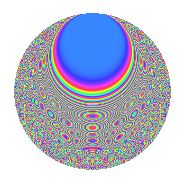# Properties

 Label 1849.2.cLevel 1849 Weight 2 Character orbit c Rep. character $$\chi_{1849}(423,\cdot)$$ Character field $$\Q(\zeta_{3})$$ Dimension 260 Sturm bound 315

# Related objects

## Defining parameters

 Level: $$N$$ $$=$$ $$1849 = 43^{2}$$ Weight: $$k$$ $$=$$ $$2$$ Character orbit: $$[\chi]$$ $$=$$ 1849.c (of order $$3$$ and degree $$2$$) Character conductor: $$\operatorname{cond}(\chi)$$ $$=$$ $$43$$ Character field: $$\Q(\zeta_{3})$$ Sturm bound: $$315$$

## Dimensions

The following table gives the dimensions of various subspaces of $$M_{2}(1849, [\chi])$$.

Total New Old
Modular forms 360 340 20
Cusp forms 272 260 12
Eisenstein series 88 80 8

## Trace form

 $$260q + 4q^{2} - 2q^{3} + 220q^{4} - 3q^{5} + 3q^{6} - q^{7} + 18q^{8} - 92q^{9} + O(q^{10})$$ $$260q + 4q^{2} - 2q^{3} + 220q^{4} - 3q^{5} + 3q^{6} - q^{7} + 18q^{8} - 92q^{9} + 7q^{10} + 12q^{11} + 2q^{12} - q^{13} + 4q^{14} - 3q^{15} + 116q^{16} + 3q^{17} + 4q^{18} + 3q^{19} - 17q^{20} - 28q^{21} - 10q^{22} + 3q^{23} - 4q^{24} - 51q^{25} - 15q^{26} + 10q^{27} + 6q^{28} + 9q^{29} - 3q^{30} + 4q^{31} + 20q^{32} + 10q^{33} + 14q^{34} + 18q^{35} - 47q^{36} - 12q^{37} - 7q^{38} + 20q^{39} + 29q^{40} + 2q^{41} + 2q^{42} - 76q^{44} - 32q^{45} - 26q^{46} + 6q^{47} - 13q^{48} - q^{49} - 17q^{50} + 22q^{51} + 16q^{52} - 11q^{53} + 48q^{54} - 31q^{56} + 3q^{57} - 6q^{58} - 14q^{59} - q^{60} - 14q^{61} + 5q^{62} - 23q^{63} - 50q^{64} + 10q^{65} - 19q^{66} - 5q^{67} - 43q^{68} - 21q^{69} - 2q^{70} + 2q^{71} + 33q^{72} + 8q^{73} - 26q^{74} - 6q^{75} + 17q^{76} + 15q^{77} - 14q^{79} - 27q^{80} + 38q^{81} + 30q^{82} + 11q^{83} + 76q^{84} - 42q^{85} - 52q^{87} - 10q^{88} + 2q^{89} - 100q^{90} - 20q^{91} + 27q^{92} + 5q^{93} + 58q^{94} - 23q^{95} + 6q^{96} + 42q^{97} + 16q^{98} - 15q^{99} + O(q^{100})$$

## Decomposition of $$S_{2}^{\mathrm{new}}(1849, [\chi])$$ into newform subspaces

The newforms in this space have not yet been added to the LMFDB.

## Decomposition of $$S_{2}^{\mathrm{old}}(1849, [\chi])$$ into lower level spaces

$$S_{2}^{\mathrm{old}}(1849, [\chi]) \cong$$ $$S_{2}^{\mathrm{new}}(43, [\chi])$$$$^{\oplus 2}$$

## Hecke characteristic polynomials

There are no characteristic polynomials of Hecke operators in the database